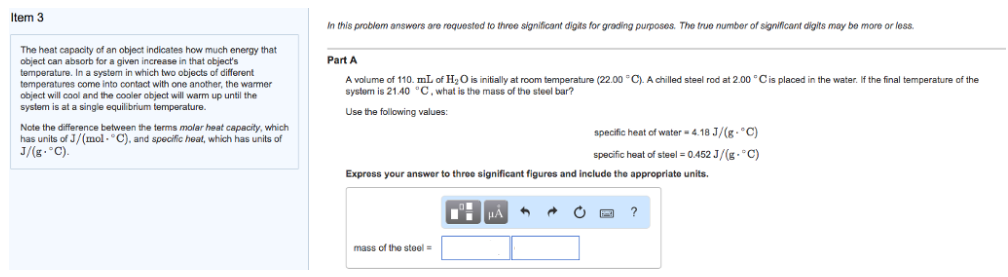# Problem: The heat capacity of an object indicates how much energy that object can absorb for a given increase in that objects temperature. In a system in which two objects of different temperatures come into contact with one another, the warmer object will cool and the cooler object will warm up until the system is at a single equilibrium temperature. Note the difference between the terms molar heat capacity, which has units of J/(mol • °C), and specific heat, which has units of J/(g • °C). A volume of 110. mL of H2O is initially at room temperature (22.00 °C). A chilled steel rod at 2.00 °C is placed in the water. If the final temperature of the system is 21.40 °C, what is the mass of the steel bar? Use the following values: specific heat of water = 4.18 J/(g • °C) specific heat of steel = 0.452 J/(g • °C) Express your answer to three significant figures and include the appropriate units.

###### FREE Expert Solution
87% (439 ratings)###### Problem Details

The heat capacity of an object indicates how much energy that object can absorb for a given increase in that objects temperature. In a system in which two objects of different temperatures come into contact with one another, the warmer object will cool and the cooler object will warm up until the system is at a single equilibrium temperature.

Note the difference between the terms molar heat capacity, which has units of J/(mol • °C), and specific heat, which has units of J/(g • °C).

A volume of 110. mL of H2O is initially at room temperature (22.00 °C). A chilled steel rod at 2.00 °C is placed in the water. If the final temperature of the system is 21.40 °C, what is the mass of the steel bar?

Use the following values:

specific heat of water = 4.18 J/(g • °C)

specific heat of steel = 0.452 J/(g • °C)

Express your answer to three significant figures and include the appropriate units.